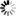Welcome to Central Library, SUST
Normal view

# A Course on Mathematical Logic [electronic resource] / by Shashi Mohan Srivastava.

Material type:TextSeries: UniversitextPublisher: New York, NY : Springer New York : Imprint: Springer, 2013Edition: 2nd ed. 2013Description: XII, 198 p. online resourceContent type:
• text
Media type:
• computer
Carrier type:
• online resource
ISBN:
• 9781461457466
Additional physical formats: Printed edition:: No titleDDC classification:
• 511.3 23
LOC classification:
• QA8.9-10.3
Online resources:
Contents:
Preface -- 1 Syntax of First-Order Logic -- 2 Semantics of First-Order Languages -- 3 Propositional Logic -- 4 Completeness Theorem for First-Order Logic -- 5 Model Theory -- 6 Recursive Functions and Arithmetization of Theories -- 7 Incompleteness Theorems and Recursion Theory -- References -- Index.
Summary: This is a short, modern, and motivated introduction to mathematical logic for upper undergraduate and beginning graduate students in mathematics and computer science. Any mathematician who is interested in getting acquainted with logic and would like to learn Gödel’s incompleteness theorems should find this book particularly useful. The treatment is thoroughly mathematical and prepares students to branch out in several areas of mathematics related to foundations and computability, such as logic, axiomatic set theory, model theory, recursion theory, and computability. In this new edition, many small and large changes have been made throughout the text.  The main purpose of this new edition is to provide a healthy first introduction to model theory, which is a very important branch of logic.  Topics in the new chapter include ultraproduct of models, elimination of quantifiers, types, applications of types to model theory, and applications to algebra, number theory and geometry.  Some proofs, such as the proof of the very important completeness theorem,  have been completely rewritten in a more clear and concise manner.  The new edition also introduces new topics, such as the notion of elementary class of structures, elementary diagrams, partial elementary maps, homogeneous structures, definability, and many more. Review from the first edition: "All results included in the book are very carefully selected and proved. The author’s manner of writing is excellent, which will surely make this book useful to many categories of readers." --Marius Tarnauceanu, Zentralblatt MATH.
Tags from this library: No tags from this library for this title.
Star ratingsAverage rating: 0.0 (0 votes)
No physical items for this record

Preface -- 1 Syntax of First-Order Logic -- 2 Semantics of First-Order Languages -- 3 Propositional Logic -- 4 Completeness Theorem for First-Order Logic -- 5 Model Theory -- 6 Recursive Functions and Arithmetization of Theories -- 7 Incompleteness Theorems and Recursion Theory -- References -- Index.

This is a short, modern, and motivated introduction to mathematical logic for upper undergraduate and beginning graduate students in mathematics and computer science. Any mathematician who is interested in getting acquainted with logic and would like to learn Gödel’s incompleteness theorems should find this book particularly useful. The treatment is thoroughly mathematical and prepares students to branch out in several areas of mathematics related to foundations and computability, such as logic, axiomatic set theory, model theory, recursion theory, and computability. In this new edition, many small and large changes have been made throughout the text.  The main purpose of this new edition is to provide a healthy first introduction to model theory, which is a very important branch of logic.  Topics in the new chapter include ultraproduct of models, elimination of quantifiers, types, applications of types to model theory, and applications to algebra, number theory and geometry.  Some proofs, such as the proof of the very important completeness theorem,  have been completely rewritten in a more clear and concise manner.  The new edition also introduces new topics, such as the notion of elementary class of structures, elementary diagrams, partial elementary maps, homogeneous structures, definability, and many more. Review from the first edition: "All results included in the book are very carefully selected and proved. The author’s manner of writing is excellent, which will surely make this book useful to many categories of readers." --Marius Tarnauceanu, Zentralblatt MATH.

There are no comments on this title.